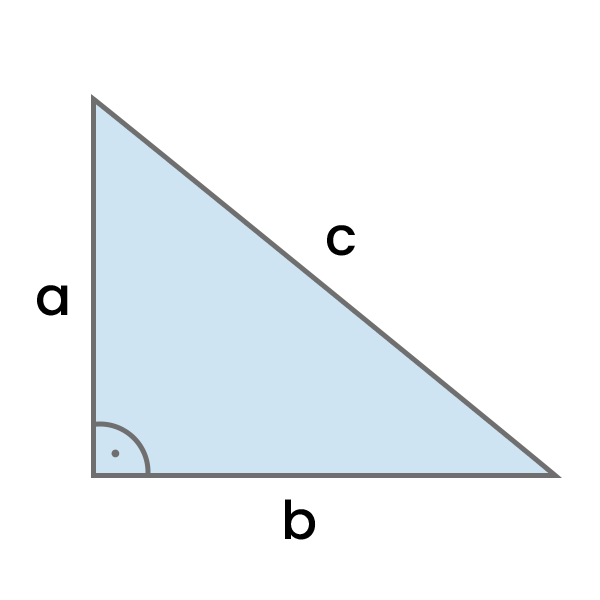# Pythagorean Theorem Calculator

Calculate the length of side a, side b, or the hypotenuse c based on the input data in your chosen units, using the Pythagorean Theorem.Result:
Calculation:
Waiting for Input...

In addition to the result and the area of the triangle, this calculator also shows the step-by-step calculation process, making it an excellent tool not only for pure calculations but also for learning.

## What is the Pythagorean Theorem?

The Pythagorean Theorem is used to calculate the length of one side of a right triangle when you know the lengths of the other two sides.

The general formula for the Pythagorean Theorem is:

$$a^{2} + b^{2} = c^{2}$$

Knowing it, we can easily calculate not only the length of the hypotenuse (c) but also, with minor modifications, the lengths of sides a or b.

## How to use this calculator?

1. Select whether you want to calculate Hypotenuse, Side A, or Side B length.
2. Select the length unit (e.g. centimeters or inches).
3. Enter both sides lengths in the specified unit.
4. Click "Calculate".
5. Check the results and step-by-step calculation process.

## Pythagorean Theorem Formula

There are several formulas we can use depending on what we want to calculate.

### Calculating the length of the Side a

Calculate the square root of the length of the hypotenuse squared minus the length of the side b squared.

$$a = \sqrt{c^{2} - b^{2}}$$

### Calculating the length of the Side b

Calculate the square root of the length of the hypotenuse squared minus the length of the side a squared.

$$b = \sqrt{c^{2} - a^{2}}$$

### Calculating the length of the Hypotenuse c

Calculate the square root of the sum of the sides squared.

$$c = \sqrt{a^{2} + b^{2}}$$

### Calculating the Area of the Right Triangle

Calculate the length of side a multiplied by the length of side b divided by 2.

$$A = \dfrac {ab}{2}$$
Math Calculators
PureTables.com
Have an idea for a tool or calculator that would make your life easier? Contact us, so we could try to create and provide it.